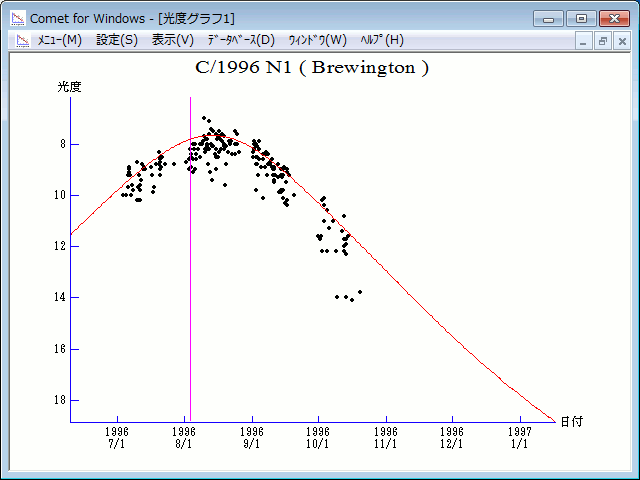# \$B%V%k!<%\$%s%H%sWB@1(B

C/1996 N1 ( Brewington )###\$B%W%m%U%#!<%k(B

 \$BH/8+F|(B 1996\$BG/(B7\$B7n(B4\$BF|(B \$BH/8+8wEY(B 10\$BEy(B \$BH/8+ Howard J. Brewington (Cloudcroft, NM)

###\$B50F;MWAG(B

```   The following improved orbital elements by Kenji Muraoka, are
from 291 observations 1996 July 4 to Nov. 3, perturbations by
9 Planets, Moon and 5 minor planets were taken into account.
The mean residual is +/- 0.74 arc seconds.

Epoch  =  1996 July 16.0  TT       JDT = 2450280.5
T  =  1996 Aug.  3.42378       +/- 0.00005 (m.e.) TT
Peri. =   43.96953                +/- 0.00009
Node  =  234.90045                +/- 0.00012   (2000.0)
Incl. =   52.14819                +/- 0.00008
q  =    0.9258217              +/- 0.0000007 AU
e  =    1.0005676              +/- 0.0000057
1/a  =   -0.0006131              +/- 0.0000062 1/AU
orig. 1/a  =   -0.0001532
fut.  1/a  =   +0.0005337
```

###\$B@1?^(B1996\$BG/(B 1\$B7n(B 8\$BF|!A(B1996\$BG/(B 8\$B7n(B15\$BF|(B1996\$BG/(B 6\$B7n(B26\$BF|!A(B1997\$BG/(B 2\$B7n(B 1\$BF|(B

###\$B8wEYJQ2=(B

```        m1 = 8.5 + 5 log\$B&\$(B + 20.0 log r(t - 12)
```##### \$B50F;MWAG\$OB<2,7r<#;a\$N7W;;\$K\$h\$k\$b\$N\$G\$9!#(B \$B@1?^\$O(B StellaNavigator Ver.2.0 for Windows (\$B%"%9%H%m%"!<%D(B \$BJTCx(B / \$B%"%9%-!<=PHG6I4)(B) \$B\$G:n@.\$7\$?\$b\$N\$G\$9!#(B \$B8wEY%0%i%U\$O(BComet for Windows\$B\$G:n@.\$7\$?\$b\$N\$G\$9!#(B